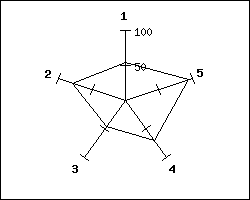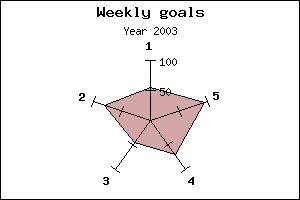Contents Previous Next

Let's start by creating a very simple radar plot based on 5 data points using mostly default values.

As the first thing you must remember to include the extension module that contains the radar plot. "jpgraph_radar.php".

A very simple radar plot is created by the code

``` <?php include ( "../jpgraph.php"); include ("../jpgraph_radar.php");      // Some data to plot \$data  = array(55,80, 46,71,95 );      // Create the graph and the plot \$graph  = new RadarGraph (250,200, "auto"); \$plot  = new RadarPlot (\$data); // Add the plot and display the graph \$graph->Add( \$plot); \$graph->Stroke(); ?>```

and would give the resultFigure 111: A first very simple radar plot using default settings [src]

To change the size and position of the radar graph is similar to the pie plot and you do it by using the methods SetSize() and SetCenter()

If you want a filled radar plot you need to specify the fill color with the method SetFillColor() The following example shows these methods in actionFigure 112: Changing size, position and adding fill color to the radar plot. [src]

Contents Previous Next